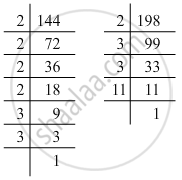# The HCF of 144 and 198 is - Mathematics

MCQ
Sum

The HCF of 144 and 198 is

• 9

• 12

• 6

• 18

#### Solution

2 × 32 = 18
We first factorise the two numbers:144 = 2 × 2 × 2 × 2 × 3 × 3 = 24 × 32
198 = 2 × 3 × 3 × 11 = 2 × 32 × 11
Here, 18 (2 × 32 = 18) is the highest common factor of the two numbers.

Concept: Highest Common Factor
Is there an error in this question or solution?

#### APPEARS IN

RS Aggarwal Class 6 Mathematics
Chapter 2 Factors and Multiples
Exercise 2F | Q 12 | Page 41